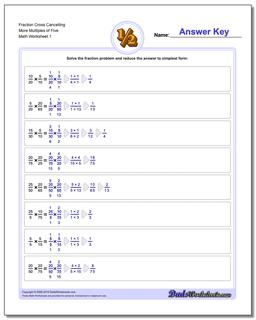# Math Worksheets: Fraction Multiplication: Fraction Multiplication: Fraction Cross Cancelling More Multiples of Five## Fraction Cross Cancelling More Multiples of Five

PropertyValue
DescriptionFraction Cross Cancelling More Multiples of Five: The multiplying fraction worksheets in this section have a heavy emphasis on problems that require you to cross multiply (or cross cancel) to get the product.
Resource TypeWorksheet